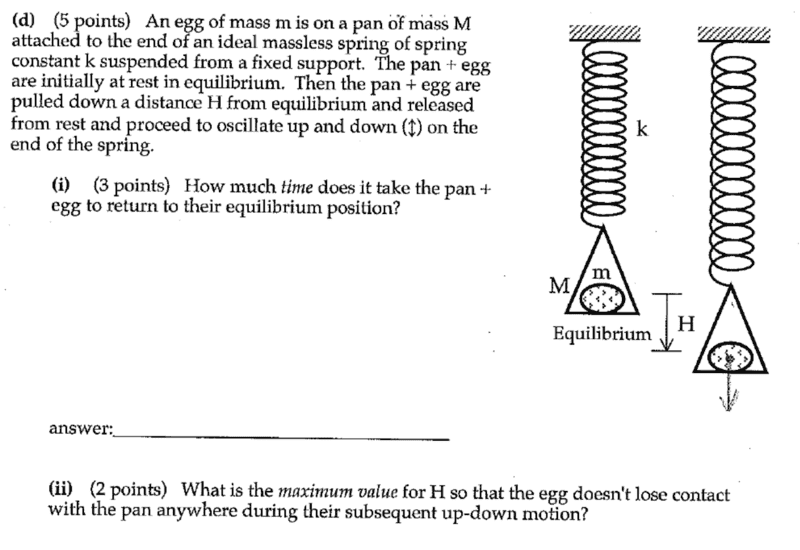# Object within a Pan Spring Motion

• srekai

## Homework Statement## Homework Equations

Spring motion equations
##\omega = \sqrt{\frac{k}{m}}##
##KE = \frac{1}{2}k \Delta x^2##
##F = -k \Delta x##
##T = \frac{1}{f}##
##f = \frac{\omega}{2 \pi}##

## The Attempt at a Solution

Part a is straightforward I believe.
Simply combine the masses as m+M, into ##\omega = \sqrt{\frac{k}{m+M}}##
So then ##T = \frac{1}{f} = \frac{2 \pi}{\sqrt{\frac{k}{m+M}}}##
I believe the time it takes to return equilibrium should half a period, so we get ##\frac{\pi}{\sqrt{\frac{k}{m+M}}}##

Part b is the tricky part, I'm not sure how one goes about it. I guess the first step would be identifying all the forces on the egg
The egg has an upwards normal force from the pan, along with a downwards force from gravity.
It would experience a force from the spring when it is being pulled up or down.
I imagine you would try to find the sum of forces and find when it is not in equilibrium?

#### Attachments

the time it takes to return equilibrium should half a period
Think that through again.
Part b is the tricky part
Consider accelerations. During SHM, where in the cycle is the maximum acceleration? What determines the maximum acceleration of the egg?

Think that through again.

Consider accelerations. During SHM, where in the cycle is the maximum acceleration? What determines the maximum acceleration of the egg?

Yeah definitely half a period, since you pass by the equilibrium point after half a period. Since spring motion is sinusoidal, it'll start from rest, into maximum amplitude, then passes by the equilibrium point, into the compressed form, back out into the equilibrium point in one period.

Acceleration is greatest at the maximum amplitudes, so either when it's fully stretched or compressed.
##a = -(2 \pi f)^2 x##

it'll start from rest, into maximum amplitude, then passes by the equilibrium point, into the compressed form, back out into the equilibrium point in one period.
Right, and the question is asking for the time from one extreme to equilibrium.
Acceleration is greatest at the maximum amplitudes, so either when it's fully stretched or compressed.
Yes. But is there anything in the set-up that limits the acceleration to which the egg can be subjected?